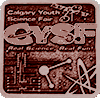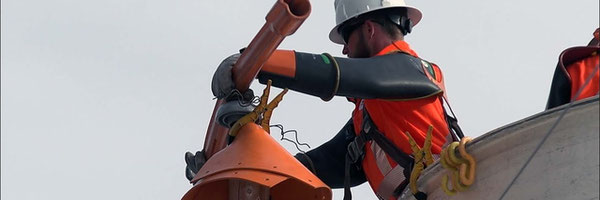HONOURABLE MENTION#### The Basel Problem: A New Way of Looking at Numbers

This project explores limits, the basel problem, facets of complex analysis, and a new way of looking at numbers.

### Problem

Objective:

• What are limits in math?
• What are their applications with solving the Basel Problem?
• Can one expand the Basel Problem into the realm of Complex Analysis? If so, how?

### Method

As I am doing a study project, I cannot fill out this section. If you would like to see my research, look below this block.

### Research

Limits (Feb 2)

• A limit is the value a function approaches as the input gets nearer to a value.
• One example of a problem that we can apply limits to is:
• (x2-1)/(x-1)
• If x=1 we can work out the equation to be 0/0 , which is indeterminate. (mathisfun)
• I do not know what I would do without Mathsisfun.com (it is a great website, and any person interested in math should go and take a look. It has been evaluated by professors around the world, and is all around amazing.)

Limits to Infinity (and beyond):

• Infinity is a concept.
• What is 1/∞ ?
• Let’s convert that equation into 1/x .
• We can see that as x increases in value, 1x   decreases in value; therefore, the limit of  1x   as x approaches infinity is 0.
• This can be written as limx→∞1x=0 .

Infinite Series:

According to “mathsisfun.com,” infinite series are “The sum of infinite terms that follow a rule.” The website then continued to give an example of an infinite series.

1/2 + 1/4 + 1/8 + 1/16 + ... = S

Solution to the Basel Problem via geometry:

The solution to the Basel Problem is π2/6 ; most people know that. However, I found a rather ground-breaking paper detailing a proof for this problem using geometry. This solution was written by Johan Wastlund of the department of Mathematics of Chalmers University. By using trigonometry, Pythagoras, and circles, the mathematician was able to prove the Basel problem relatively easily. The proof uses trigonometry and circles to prove Euler’s solution. Imagine a lake with stars around it with you, an observer at a point on the circumference of the lake. Say that you receive a total brightness of  π2/4  from the stars. How can we increase the number of stars without decreasing the amount of brightness? We have two things that can help us: the inverse square law and the obscure inverse Pythagorean theorem. Essentially, by using these two tools, we can double the number of stars without decreasing the amount of brightness. As the number of stars approaches infinity, the circle becomes bigger (to a diameter, radius, and circumference of infinity), the limit, as mathematician Ben Hambrecht tells us, (from the observer’s point of view), becomes a line. Then, we cut out the negative numbers in this circular number line, and we get π2/8 . As the sum only includes positive odd numbers, we multiply the fraction by 4 thirds, giving us the grand total of π2/6.

### Data

For my study project in particular, this is my analysis on the data.

From the above conjecture, one can surmise that, as the mathematician said: “The number line is a limit of an infinitely large circle.” (Hambrecht) Then, it is also assumed that all the stars along the circumference of this circle have the same amount of brightness. After that, it is also assumed that due to the application of the inverse square law, the circle is in a 3-dimensional space, with the stars and the circle like sheets of paper with a height of zero, and the observer being at the point of tangency on an imaginary tangent line.

With this view that the number line is a circle, we can incorporate complex analysis by asking where imaginary numbers can be incorporated within this structure. One way that we can do this is by incorporating a Riemann sphere, satisfying the need to incorporate lateral or complex numbers on the number circle. However, this would imply that imaginary numbers are on the number line, which is patently false. On the other hand, one might assume that as the circle is 2-dimesional and the imaginary components are the depth of the 2 dimensional circle; this would satisfy all the conditions we have placed upon ourselves while implying that the number line is a 2-dimensional projection of the Riemann Sphere. This, furthermore, implies that the number line is a slice of the infinitely large Riemann Sphere, further implying that there are infinite infinite number lines.

### Conclusion

In short, based on the two aforementioned conjectures, one can assume that the number line is a projection of a Riemann Sphere. Furthermore, the number line that we know is simply a slice of the Riemann sphere. Limits are the value a function approaches as the input gets nearer to a value. Limits’ applications with the Basel problem are in finding the limit of an infinitely large circle in which all the inverse squares of all the odd numbers are contained, then cutting all the negative odd numbers and multiplying by 4/3 to find the ultimate answer: π2/6 .

### Acknowledgement

I would like to acknowledge my amazing math teacher, Jaclyn Roulston, for (very patiently) answering all of my questions pertaining to any area of math. I would also like to thank the organizers of my school's science fair, Heather Lai, Scott Ferg, and Laura Cruickshank for allowing students at our school to participate in this event. Finally, I would like to thank you, the judge, for taking some precious time out of your day and judging my Science Fair project.